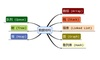• 用栈实现计算器（数据结构作业） 用栈实现一个计算器，计算器的功能包括： 1.加减乘除四则运算 2.含括号的运算 3.小数乘法和除法运算 以下是我的代码，很难找到比我这个还短的代码了吧～(￣▽￣～) #include<bits...
用栈实现计算器（数据结构作业）
用栈实现一个计算器，计算器的功能包括：
1.加减乘除四则运算
2.含括号的运算
3.小数乘法和除法运算
以下是我的代码，很难找到比我这个还短的代码了吧～(￣▽￣～)
#include<bits/stdc++.h>
using namespace std;
int cmp(char c)
{
if(c == '-' || c == '+') return 1;
if(c == '*' || c == '/') return 2;
return 0;
}
void compute(stack<double> &N, stack<char> &T)
{
double b = N.top();
N.pop();
double a = N.top();
N.pop();
switch(T.top())
{
case'+': N.push(a + b); break;
case'-': N.push(a - b); break;
case'*': N.push(a * b); break;
case'/': N.push(a / b); break;
}
T.pop();
}
int main()
{
string c;
stack<double> N;
stack<char> T;
printf("请输入算术表达式,并以=结束.\n");
while(cin >> c)
{
int len = c.length();
for(int i = 0; i < len; i++)
{
if(isdigit(c[i]))
{
double number = atof(&c[i]);
while(i < len && (isdigit(c[i]) || c[i] == '.')) i++;
i--;               //回到当前字符
N.push(number);
}
else
{
if(c[i] == '(') T.push(c[i]);	//左括号直接入栈
else if(c[i] == ')')  //右括号 出栈计算直到左括号为止
{
while(T.top() != '(') compute(N, T);
T.pop();
}
else if(T.empty() || cmp(c[i]) > cmp(T.top())) T.push(c[i]);
//栈空或者当前字符比栈顶字符优先级要高 ，直接入栈
else //当前字符比栈顶字符优先级要低或者级别相同，那就出栈计算
{
while(!T.empty() && cmp(c[i]) <= cmp(T.top())) compute(N, T);
T.push(c[i]);
}
}
}
T.pop();
printf("%.8lf\n", N.top());
N.pop();
}
return 0;
}

原创不易，如果觉得有用，欢迎点赞收藏关注我^ _ ^
如果有不懂的欢迎评论提出(◕ܫ◕)


展开全文计算器 括号匹配
• 谁要的数据结构作业? 举神和ww经常在一起玩关于石子的游戏，今天他们又发明了一种新的玩法，每次他们两个可以把两堆石子合并得到一堆更重的石子，但需要消耗与这两堆石子相同重量的体力。 比如:举神和ww把重量为3和...
谁要的数据结构作业?

举神和ww经常在一起玩关于石子的游戏，今天他们又发明了一种新的玩法，每次他们两个可以把两堆石子合并得到一堆更重的石子，但需要消耗与这两堆石子相同重量的体力。 比如:举神和ww把重量为3和5的石子堆合并，可以得到一个重量为8的石子堆，但同时需要消耗3+5的体力值。 现在他两想把n堆石子合并到只剩下一堆，但他们又不想消耗太多体力，你能帮他们求出消耗的最少体力吗？ 数据范围:n<=20000

Input

第一行为一个整数n，表示开始时石子的总堆数 ，接下来n行，每行一个整数a[i] (1<=a[i]<=50000) 代表开始时每堆石子的重量。

Output

输出包含一个整数，表示他们消耗的最小体力。

Sample Input

3
8
5
8

Sample Output

34

AC代码：优先队列知识戳💨队列及优先队列及栈简单用法
#include<iostream>
#include<queue>//头文件
using namespace std;
int main()
{
int n,a,h;
long long sum=0;//不定义成long long 会Wrong Answer
cin>>n;
priority_queue<int,vector<int>,greater<int> >p;//从小到大排序
while(n--)
{
cin>>a;
p.push(a);
}
while(p.size()>1)
{
h=p.top();
p.pop();
h=h+p.top();
p.pop();
p.push(h);
sum=sum+h;
}
cout<<sum<<endl;
return 0;
}


永远相信美好🎈


展开全文队列 c++
• 数据结构作业 栈 实现 括号匹配 问题
数据结构作业  栈  实现 括号匹配 问题
毕竟太菜 照着demo敲了几天才 搞定。
栈的几个基本应用函数顺便敲了  其他的就不详说了.
见代码：
#include <stdio.h>
#include <stdlib.h>

#define OK      1
#define ERROR   0
#define TRUE    1
#define FALSE   0
#define OVERFLOW -1
#define STACK_INIT_SIZE 100
#define STACKINCREMENT 10
#define BUFFERSIZE 200

typedef int Status; //函数返回状态
typedef char SElemType;  //栈元素类型
typedef struct{//栈结构定义
SElemType *base;
SElemType *top;
int stacksize;
}SqStack;

Status InitStack(SqStack *S)
{
//构造一个空栈S
S->base=(SElemType *)malloc(STACK_INIT_SIZE*sizeof(SElemType));
if(!S->base)//分配失败
{
printf("分配内存失败.\n");
return 0;
}
S->top=S->base;
S->stacksize=STACK_INIT_SIZE;
return OK;
}

Status DestroyStack(SqStack *S)
{
//销毁栈S，S不再存在
if(!S)//S为空
{
printf("指针为空，释放失败.\n");
exit(0);
}
free(S);
return OK;
}

Status ClearStack(SqStack *S)
{
//把栈S置为空栈
if(!S)//S不存在
return FALSE;
S->top=S->base;//直接将栈顶指针指向栈底
return OK;
}

Status StackEmpty(SqStack S)
{
//若栈S为空栈，则返回TRUE，否则返回FALSE
if(S.top==S.base)
return TRUE;
else
return FALSE;
}

int StackLength(SqStack S)
{
//返回S元素的个数，即栈的长度
return S.stacksize;
}

Status GetTop(SqStack S,SElemType *e)
{
//若栈不为空，则用e返回S的栈顶元素，并返回OK；否则返回FALSE
if(S.top==S.base)
{
return FALSE;
}
else
{
*e=*(S.top-1);
return OK;
}
}

Status Push(SqStack *S,SElemType e)
{
//插入元素e为新的栈顶元素
if(S->top-S->base>=S->stacksize)//栈已满，追加存储空间
{
S->base=(SElemType *)realloc(S->base,(S->stacksize+STACKINCREMENT)*sizeof(SElemType));
if(!S->base)
{
printf("重新申请空间失败.\n");
exit(0);
}
S->top=S->base+S->stacksize;//更改栈顶指针
S->stacksize+=STACKINCREMENT;
}
*S->top++=e;
return OK;
}

Status Pop(SqStack *S,SElemType *e)
{
//若栈S不为空，则删除S的栈顶元素，用e返回其值，并返回OK,否则返回ERROR
if(S->top==S->base)//栈为空
{
printf("栈为空.\n");
return ERROR;
}
*e=*(--S->top);
return OK;
}

Status StackTraverse(const SqStack *S){
//从栈底到栈顶依次对每个元素进行访问
SElemType *p=S->base;
if(S->base==S->top)
{
printf("栈为空.\n");
return FALSE;
}
while(p!=S->top)
{
printf("%c",*p++);
}
printf("\n");
return OK;
}

Status BracketMatch(SqStack *S,const char *string){
//输入字符串string中的括号匹配，返回TRUE；否则返回FALSE
const char *p=string;
SElemType e;
InitStack(S);
while(*p!='\0')//遍历字符串
{
switch(*p)//判断p的值
{
case '('://左括号，入栈
case '[':
case '{':
Push(S,*p);
break;
case ')':
if(FALSE==GetTop(*S,&e))
return FALSE;
if(e=='(')
{
if(ERROR==Pop(S,&e))
return FALSE;
}
else
return FALSE;
break;
case ']':
if(FALSE==GetTop(*S,&e))
return FALSE;
if(e=='[')
{
if(ERROR==Pop(S,&e))
return FALSE;
}
else
return FALSE;
break;
case '}':
if(FALSE==GetTop(*S,&e))
return FALSE;
if(e=='{')
{
if(ERROR==Pop(S,&e))
return FALSE;
}
else
return FALSE;
break;
default:  ;
}
p++;
}
if(!StackEmpty(*S)) //字符串遍历完，栈非空，不匹配
return FALSE;
return TRUE;
}

int main()
{
char *String;
SqStack stack;
String=(char*)malloc(sizeof(char)*BUFFERSIZE);
if(!String)
{
printf("分配内存失败.\n");
return 0;
}
while(1)
{
printf("请输入一行含括号的表达式（输入\"!\"退出）:");
gets(String);
if(String=='!')//退出
break;
if(TRUE==BracketMatch(&stack,String))
{
printf("\n能正确匹配.\n\n");
}
else
{
printf("\n不能正确匹配.\n\n");
}
}
return 0;
}

展开全文• 数据结构作业，哈夫曼树的创建，编码，解码 哈夫曼树的生成： #define M 80 typedef struct { char data; int weight; int parent,lch,rch; }NodeType; typedef NodeType HufTree[M+1]; typedef char ** ...
数据结构作业，哈夫曼树的创建，编码，解码
哈夫曼树的生成：

#define M 80
typedef struct
{
char data;
int weight;
int parent,lch,rch;
}NodeType;
typedef NodeType HufTree[M+1];
typedef char ** HufCode;
/*在huffman树中选出两个双亲为0且权值最小的结点*/
void select(HufTree ht,int n,int *s1,int *s2)
{
int i,j;int t=1000;
for(i=1;i<=n;i++)
{
if(ht[i].parent==0&&ht[i].weight<=t)
{
*s1=i;
t=ht[i].weight;
}
}
t=1000;
for(j=1;j<=n;j++)
{
if(ht[j].parent==0&&ht[j].weight<=t&&j!=*s1)
{
*s2=j;
t=ht[j].weight;
}
}
}
/*生成huffman树*/
void huf_tree(float w[],char data[],HufTree &ht,int n)
{
int i;int s1,s2;
for(i=1;i<=n;i++)
{
ht[i].weight=w[i-1];
ht[i].data=data[i-1];
ht[i].parent=0;
ht[i].lch=0;ht[i].rch=0;
}
for(i=n+1;i<=2*n-1;i++)
ht[i].parent=0;
for(i=n+1;i<=2*n-1;i++)
{
select(ht,i-1,&s1,&s2);
ht[i].weight=ht[s1].weight+ht[s2].weight;
ht[i].lch=s2;ht[i].rch=s1;
ht[s1].parent=i;ht[s2].parent=i;
}
}

哈夫曼树的编码：
void huf_code(HufCode &hcd,HufTree ht,int n)
{
hcd=(HufCode)malloc(sizeof(char *)*(n+1));
char *cd;
cd=(char *)malloc(sizeof(char)*n);
int i;
for(i=1;i<=n;i++)
{
cd[n-1]='\0';
int start;
start=n-1;int c=i;
int f=ht[c].parent;
while(f!=0)
{
if(ht[f].lch==c)
cd[--start]='0';
else
cd[--start]='1';
c=f;f=ht[c].parent;
}
hcd[i]=(char *)malloc(sizeof(char)*(n-start));
strcpy(hcd[i],&cd[start]);
}
}
int search(char data[],HufCode hcd,int n)
{
int i,t=0;
for(i=1;i<=n;i++)
{
if(strcmp(hcd[i],data)==0)
{
t=i;
break;
}
}
return t;
}


哈夫曼树的解码：
void huf_decode(HufCode hcd,HufTree ht,char code[],int n)
{
char data;
int i=0,j=0;int t;
while(code[i]!='\0')
{
data[j]=code[i];
data[j+1]='\0';
if((t=search(data,hcd,n))!=0)
{
printf("%c",ht[t].data);
j=0;i++;
}
else
{
i++;j++;
}
}
}




展开全文• 2-1在数据结构中，与所使用的计算机无关的数据结构是（）。 (2分) A.逻辑结构和存储结构 B.物理结构 C.存储结构 D.逻辑结构 作者: 严冰 单位: 浙江大学城市学院 2-2以下说法正确的是（ ）。 (1分) A.一些...
• 一个堆栈中先后有1 2 3 4入栈，请问可能的出栈序列？数据结构作业，谢谢
• 介绍学习了数据结构课程中的一些简单的数据结构之后，用C语言和数据结构中的一些知识实现一个简易的学校的导航系统。 基本上的功能如下：数据结构采用图来存储信息，根据百度地图抽象出图的结构使用的是邻接矩阵，...c语言
• ## 数据结构作业-3

千次阅读 2011-03-22 17:30:00
设线性表为顺序存储结构。 1．需求分析 将非递减有序的单链表中值相同的多余结点删除，设线性表为顺序存储结构。 当用户输入的数组的关键码为非递减有序时，删除重复节点；当用户输入的数组的关键码并不是有序时...delete list struct
• 设待排序数据元素的关键字为：65,78,21,30,80,7,79,57,35,26，请按照下列算法对这组数据元素按关键字升序排序（以教材所给出算法为标准），给出每个算法的前2趟排序结果。 注意：不要写错关键字造成扣分，比如35写成...算法 排序算法 快速排序 堆排序
• 说明：这是数据结构学完栈和队列写的作业。利用随机数生成一张迷宫图，然后用队列找出最短路径，用栈输出之。可以动态显示最短路径喔~~~最后，这个作业用了文件分离写（头文件声明，.cpp文件定义）。 Last but not ...
• 问题及代码： 编写一个程序，求解背包问题：设有不同价值、不同重量的物品n件，求从这n件物品中选取一部分物品的方案，使选中物品的总重量不超过指定的限制重量，但选中物品的总价值最大。 /*烟台大学计算机学院 ...
• 1 ( 10分 ) 对于图中的有向图： （1）请给出每个顶点的入度； （2）请给出邻接矩阵（以教材为准）； （3）请画出所有强连通分量。 2 ( 10分 ) 画出图中的无向图的邻接矩阵。...给定一个只有7个顶点（顶点从0开始编号...链表 算法
• 实现一个哈夫曼编码系统，系统包括以下功能： 字符信息统计：读取待编码的源文件SourceFile.txt，统计出现的字符及其频率。 建立哈夫曼树：根据统计结果建立哈夫曼树。 建立哈夫曼码表：利用得到的哈夫曼树，将各...
• # Type 为数据类型, Container 为保存数据的容器, Functional 为元素比较方式 # Container 默认用的是 vector, Functional 默认用 operator< # 默认形成大顶堆, 队头元素最大 # 上面程序中传入 compare 比较函数, ...c 快速
• 数据结构* 单链表、C逆序、C两个递增的链表合并成一个递增的链表题目地址 MOOC 请完成下列算法填空实现对顺序表逆置存储，逆置存储是指将元素线性关系逆置后的顺序存储，例如（a0,a1,a2），关系逆置后为（a2,a1,a0)....
• 2-6为解决计算机主机与打印机之间速度不匹配问题，通常设置一个打印数据缓冲区，主机将要输出的数据依次写入该缓冲区，而打印机则依次从该缓冲区中取出数据。该缓冲区的逻辑结构应该是？ (1分) A.树 B.队列 ...
• 2-1数据结构中Dijkstra算法用来解决哪个问题？ (1分) A.字符串匹配 B.最短路径 C.拓扑排序 D.关键路径 作者: DS课程组 单位: 浙江大学 2-2若要求在找到从S到其他顶点最短路的同时，还给出不同的最短路的条数...
• 数据结构：邻接矩阵// xydy.cpp : 定义控制台应用程序的入口点。 // //#include "stdafx.h" #include "iostream" #include "cstdio" #include "string" #include "cstring" #include "stack" #define N 100 #define ...
• 1-2不论是入队列操作还是入栈操作,在顺序存储结构上都需要考虑"溢出"情况。 (2分) T F 作者: 林华 单位: 广东外语外贸大学 1-3在用数组表示的循环队列中，front值一定小于等于rear值。 (1分) T F...
• 2-10线性表若采用链式存储结构时，要求内存中可用存储单元的地址 (1分) A.连续或不连续都可以 B.一定是不连续的 C.部分地址必须是连续的 D.必须是连续的 作者: DS课程组 单位: 浙江大学 2-...
• 2-5线性表若采用链式存储结构时，要求内存中可用存储单元的地址 (1分) A.部分地址必须是连续的 B.一定是不连续的 C.连续或不连续都可以 D.必须是连续的 作者: DS课程组 单位: 浙江大学 2-6...
• #include <iostream> #define MAXSIZE 100 using namespace std; typedef char TElemType; typedef struct BiTNode { TElemType data;... struct BiTNode *lchild,*rchild;...void InOrderTr...遍历
• 2-1令P代表入栈，O代表出栈。若利用堆栈将中缀表达式3*2+8/4转为后缀表达式，则相应的堆栈操作序列是： (3分) A.PPPOOO B.POPOPO C.POPPOO D.PPOOPO 作者: DS课程组 单位: 浙江大学 ...2-2若借助堆栈将中缀...
• 6-3 修改学生成绩 （15 分） 输入n(n&lt;50)个学生的成绩信息，再输入一个学生的学号、课程以及成绩，在自定义函数update_score()中修改该学生指定课程的成绩。...其中p是结构指针，n是学生个数，co...
• 2-1给定有权无向图如下。关于其最小生成树，下列哪句是对的？ (3分) A.边(B, F)一定在树中，树的总权重为23 B.边(H, G)一定在树中，树的总权重为20 C.最小生成树唯一，其总权重为20 D.... A.......

# 数据结构作业数据结构 订阅